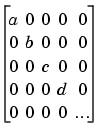MATLAB Function Referenceblkdiag

Construct a block diagonal matrix from input arguments

Syntax

• ```out = blkdiag(a,b,c,d,...)
```

Description

```out = blkdiag(a,b,c,d,...) ``` , where `a`, `b`, `c`, `d`, `...` are matrices, outputs a block diagonal matrix of the form

•The input matrices do not have to be square, nor do they have to be of equal size.

See Also

`diag`, `horzcat`, `vertcat`

© 1994-2005 The MathWorks, Inc.